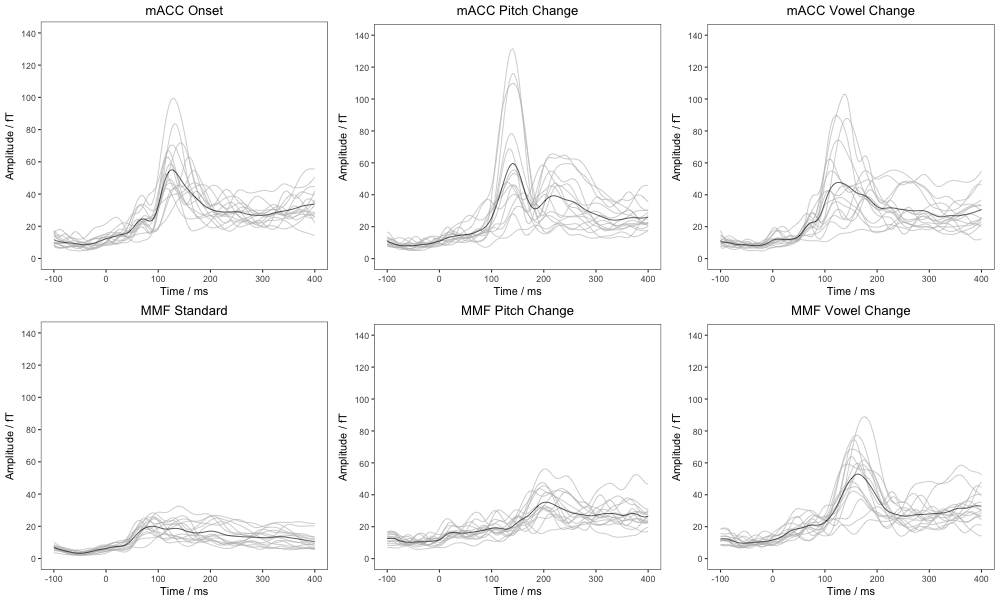``````library(ez)
library(ggplot2)
library(knitr)
library(ggplot2)
library(gridExtra)
library(plyr)
sessionInfo()``````
``````## R version 3.3.2 (2016-10-31)
## Platform: x86_64-apple-darwin13.4.0 (64-bit)
## Running under: macOS Sierra 10.12.2
##
## locale:
##  en_AU.UTF-8/en_AU.UTF-8/en_AU.UTF-8/C/en_AU.UTF-8/en_AU.UTF-8
##
## attached base packages:
##  stats     graphics  grDevices utils     datasets  methods   base
##
## other attached packages:
##  plyr_1.8.4      gridExtra_2.2.1 knitr_1.15.1    ggplot2_2.2.1
##  ez_4.4-0
##
## loaded via a namespace (and not attached):
##   Rcpp_0.12.8        nloptr_1.0.4       tools_3.3.2
##   digest_0.6.11      lme4_1.1-12        evaluate_0.10
##   tibble_1.2         nlme_3.1-128       gtable_0.2.0
##  lattice_0.20-34    mgcv_1.8-15        Matrix_1.2-7.1
##  yaml_2.1.14        parallel_3.3.2     SparseM_1.74
##  stringr_1.1.0      MatrixModels_0.4-1 rprojroot_1.1
##  grid_3.3.2         nnet_7.3-12        rmarkdown_1.3
##  minqa_1.2.4        reshape2_1.4.2     car_2.1-4
##  magrittr_1.5       backports_1.0.4    scales_0.4.1
##  htmltools_0.3.5    MASS_7.3-45        splines_3.3.2
##  assertthat_0.1     pbkrtest_0.4-6     colorspace_1.3-2
##  quantreg_5.29      stringi_1.1.2      lazyeval_0.2.0
##  munsell_0.4.3``````

# Global field power

``````GFPs <- read.csv("output/data/GFPs.csv", stringsAsFactors=TRUE)
str(GFPs)``````
``````## 'data.frame':    24570 obs. of  6 variables:
##  \$ X        : int  1 2 3 4 5 6 7 8 9 10 ...
##  \$ time     : int  -100 -96 -92 -88 -84 -80 -76 -72 -68 -64 ...
##  \$ Response : Factor w/ 13 levels "Offset","Onset",..: 2 2 2 2 2 2 2 2 2 2 ...
##  \$ Amplitude: num  8.53 7.66 7.23 6.76 6.62 ...
##  \$ SubNum   : Factor w/ 15 levels "S019","S392",..: 1 1 1 1 1 1 1 1 1 1 ...
##  \$ Paradigm : Factor w/ 2 levels "ACC","MMF": 1 1 1 1 1 1 1 1 1 1 ...``````

Define the GFP_Plot function. This plots the global field power for a specified response, taking as input the GFPs dataframe. It plots the GFP for each individual participant and overlays the grand mean (average across participants).

``````GFP_Plot <- function (Response, Title="") {
require(plyr)
require(ggplot2)
IndGFPs <- GFPs[(GFPs\$Response==Response),]
IndGFPs\$X <- NULL
GM_GFP <- ddply(IndGFPs, .(Paradigm, Response, time), summarize, Amplitude = mean(Amplitude))
GM_GFP\$SubNum <- "ZZZ" # Ensures that grand mean is plotted last (ie on top of individual participants)
GM_GFP\$Colour <- "a"
IndGFPs\$Colour <- "b"
PlotData <- rbind(IndGFPs, GM_GFP)
myPlot <- ggplot(data=PlotData, aes(x = time, y = Amplitude, group = SubNum, colour = Colour)) +
geom_freqpoly(stat = "identity", size = I(.3)) +
ggtitle(Title) +
scale_y_continuous("Amplitude / fT",limits=c(0,140),breaks=seq(0, 140, 20)) +
scale_x_continuous("Time / ms",limits=c(-100,400),breaks=seq(-100, 400, 100)) +
theme_bw() +
theme(plot.title = element_text(hjust = 0.5), legend.position="none", axis.line = element_blank(), panel.grid.major = element_blank(), panel.grid.minor = element_blank(), panel.background = element_blank()) +
scale_colour_manual(values=c("black", "grey"))
myPlot
}``````

Apply the GFP_Plot function to each response.

``````GFP_ACC_Onset <- GFP_Plot ("Onset", Title="mACC Onset")
GFP_ACC_Pitch <- GFP_Plot ("Pitch.Up", Title="mACC Pitch Change")
GFP_ACC_Vowel <- GFP_Plot ("Vowel.EU", Title="mACC Vowel Change")
GFP_MMF_Standard <- GFP_Plot ("standard", Title ="MMF Standard")
GFP_MMF_Pitch <- GFP_Plot ("Pitch.MMF", Title="MMF Pitch Change")
GFP_MMF_Vowel <- GFP_Plot ("Vowel.MMF", Title="MMF Vowel Change")``````

Combine into a six panel figure and output as a PNG file. This is Figure 2 in the paper.

``````png(filename="output/figures/Fig2.png", width=1000, height=600)
grid.arrange (GFP_ACC_Onset, GFP_ACC_Pitch, GFP_ACC_Vowel, GFP_MMF_Standard, GFP_MMF_Pitch, GFP_MMF_Vowel, ncol=3, nrow =2, widths=c(2.3, 2.3, 2.3), heights=c(2.3, 2.3))
dev.off()``````
``````## quartz_off_screen
##                 2``````
``knitr::include_graphics("output/figures/Fig2.png")``# Source waveforms

``````SWFs <- read.csv("output/data/SWFs.csv", stringsAsFactors=TRUE)
str(SWFs)``````
``````## 'data.frame':    49140 obs. of  7 variables:
##  \$ X         : int  1 2 3 4 5 6 7 8 9 10 ...
##  \$ time      : int  -100 -96 -92 -88 -84 -80 -76 -72 -68 -64 ...
##  \$ Response  : Factor w/ 13 levels "Offset","Onset",..: 2 2 2 2 2 2 2 2 2 2 ...
##  \$ Amplitude : num  -2.97 -1.79 -1.08 -1.07 -1.7 ...
##  \$ SubNum    : Factor w/ 15 levels "S019","S392",..: 1 1 1 1 1 1 1 1 1 1 ...
##  \$ Paradigm  : Factor w/ 2 levels "ACC","MMF": 1 1 1 1 1 1 1 1 1 1 ...
##  \$ Hemisphere: Factor w/ 2 levels "L","R": 1 1 1 1 1 1 1 1 1 1 ...``````

Define the SWF_Plot function. This plots the left and right source waveforms for a specified response, taking as input the SWFs dataframe. It plots the SWF for each individual participant and overlays the grand mean (average across participants).

``````SWF_Plot <- function (Response, Title="") {
require(plyr)
require(ggplot2)
IndSWFs <- SWFs[(SWFs\$Response==Response),]
IndSWFs\$X <- NULL
GM_SWF <- ddply(IndSWFs, .(Paradigm, Response, Hemisphere, time), summarize, Amplitude = mean(Amplitude))
GM_SWF\$SubNum <- "ZZZ"
GM_SWF\$Colour <- "a"
IndSWFs\$Colour <- "b"
PlotData <- rbind(GM_SWF, IndSWFs)
myPlot <- ggplot(data=PlotData, aes(x = time, y = Amplitude, group = SubNum, colour = Colour)) +
geom_freqpoly(stat = "identity", size = I(.3)) +
facet_grid(.~Hemisphere)+
ggtitle(Title) + theme(plot.title = element_text(size = 16, hjust = 0.2, vjust = 0.8)) +
scale_y_continuous("Amplitude",limits=c(-550,250),breaks=seq(-500, 200, 100)) +
scale_x_continuous("Time / ms",limits=c(-100,400),breaks=seq(-100, 400, 100)) +
theme_bw() +
theme(plot.title = element_text(hjust = 0.5), legend.position="none", axis.line = element_blank(), panel.grid.major = element_blank(), panel.grid.minor = element_blank(), panel.background = element_blank()) +
theme(strip.background = element_blank()) +
scale_colour_manual(values=c("black", "grey"))
myPlot
}``````

Apply the GFP_Plot function to each response.

``````ACC_Onset <- SWF_Plot ("Onset", Title="mACC Onset")
ACC_Pitch <- SWF_Plot ("Pitch.Up", Title="mACC Pitch Change")
ACC_Vowel <- SWF_Plot ("Vowel.EU", Title="mACC Vowel Change")
MMF_Standard <- SWF_Plot ("standard", Title ="MMF Standard")
MMF_Pitch <- SWF_Plot ("Pitch.MMF", Title="MMF Pitch Change")
MMF_Vowel <- SWF_Plot ("Vowel.MMF", Title="MMF Vowel Change")``````

Combine into a six panel figure.

``````png(filename="output/figures/Fig3_temp.png", width=1000, height=600)
grid.arrange (ACC_Onset, ACC_Pitch, ACC_Vowel, MMF_Standard, MMF_Pitch, MMF_Vowel, ncol=3, nrow =2, widths=c(5, 5, 5), heights=c(2.3, 2.3))
dev.off()``````
``````## quartz_off_screen
##                 2``````
``knitr::include_graphics("output/figures/Fig3_temp.png")``